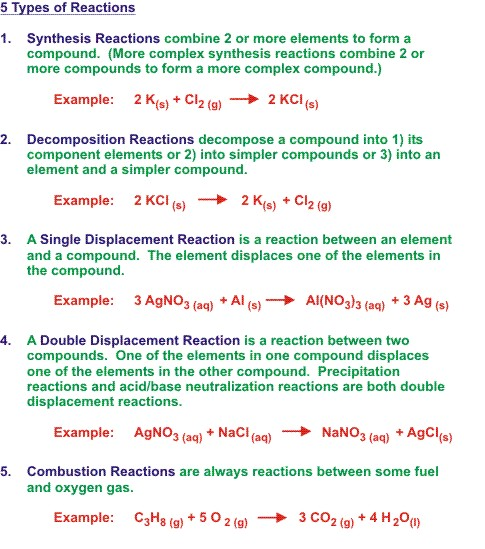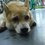# chemical reactions and the equationsChemical Reaction ~ The transformation of chemical substance into another chemical substance is known as Chemical Reaction.

Example: rusting of iron, setting of milk into curd and others.

In chemical reaction NEW SUBSTANCE is formed which is completely different in properties from the original substance, so in a chemical reaction chemical change takes place.

Following are the signs of chemical reaction:

(a) Change of state of substance.

(b) Change of colour of substance.

(c) Evolution of heat.

(d) Absorption of heat.

(e) Evolution of gas.

(f) Evolution of light.

(g) Evolution of sound,

                            Reactant and Product:


Reactant~Substances which take part in a chemical reaction are called reactants.

Product~New substance formed after a chemical reaction is called product.

Chemical Equation ~Representation of chemical reaction using symbols of substances is called chemical equation.

Example: A+B 　－－－＞　C+D

In this equation, A and B are called reactants and C and D are called the products. Arrow shows the direction of chemical reaction. Condition, if any, is written generally above the arrow.

When hydrogen reacts with oxygen, it gives water. This reaction can be represented by following chemical equation. H2 + O2 ------------> H2O

In first equation words are used and in second symbols of substances are used to write the chemical equation. For convenience, symbol of substance is used to represent chemical equations.

Chemical Equation is a way to represent the chemical reaction in concise and informative way.

Chemical equation can be divided into two types ~ Balanced Chemical Equation ~Unbalanced Chemical Equation.

                              Balanced Chemical Equation


~A balanced chemical equation has number atoms of each element equal on both sides.

Example: In this equation, numbers of zinc, hydrogen and sulphate are equal on both sides, so it is a BALANCED chemical equation.

Zn(s) + H2SO4(aq) ---> ZnSO4 (s)+ H2 (g)

According to Law of Conservation of Mass, mass can neither be created nor destroyed in a chemical reaction. To obey this law, the total mass of elements present in reactants must be equal to the total mass of elements present in products.

                                   Unbalanced Chemical Equation


~ If the number of atoms of each element in reactants is not equal to the number of atoms of each element present in product, then the chemical equation is called unbalanced chemical equation.

Example: Fe(s) + H2O(l) ----> FE3O4 (s)+ H2 (g)

In this example number atoms of elements are not equal on two sides of the reaction. For example, on the left hand side only one iron atom is present, while three iron atoms are present on the right hand side. Therefore, it is an UNBALANCED chemical equation.Note by Nicole Ling
7 years, 6 months ago

This discussion board is a place to discuss our Daily Challenges and the math and science related to those challenges. Explanations are more than just a solution — they should explain the steps and thinking strategies that you used to obtain the solution. Comments should further the discussion of math and science.

When posting on Brilliant:

• Use the emojis to react to an explanation, whether you're congratulating a job well done , or just really confused .
• Ask specific questions about the challenge or the steps in somebody's explanation. Well-posed questions can add a lot to the discussion, but posting "I don't understand!" doesn't help anyone.
• Try to contribute something new to the discussion, whether it is an extension, generalization or other idea related to the challenge.

MarkdownAppears as
*italics* or _italics_ italics
**bold** or __bold__ bold
- bulleted- list
• bulleted
• list
1. numbered2. list
1. numbered
2. list
Note: you must add a full line of space before and after lists for them to show up correctly
paragraph 1paragraph 2

paragraph 1

paragraph 2

[example link](https://brilliant.org)example link
> This is a quote
This is a quote
    # I indented these lines
# 4 spaces, and now they show
# up as a code block.

print "hello world"
# I indented these lines
# 4 spaces, and now they show
# up as a code block.

print "hello world"
MathAppears as
Remember to wrap math in $$ ... $$ or $ ... $ to ensure proper formatting.
2 \times 3 $2 \times 3$
2^{34} $2^{34}$
a_{i-1} $a_{i-1}$
\frac{2}{3} $\frac{2}{3}$
\sqrt{2} $\sqrt{2}$
\sum_{i=1}^3 $\sum_{i=1}^3$
\sin \theta $\sin \theta$
\boxed{123} $\boxed{123}$

Sort by:

thats good give me more high

- 7 years, 6 months ago

- 7 years, 6 months ago

Very nice!

- 7 years, 6 months ago

why is there something not on oxidation reactions

- 7 years, 6 months ago

Ah, those reactions are a bit more in-depth in chemistry, like hydrolysis reaction or isomeration etc. Something I would like to add is a neutralisation reaction.

Neutralisation reaction is when you combine an acid and a base. Acid + Base $\rightarrow$ Salt + Water.

Even so, there are still other smaller categories... But these are, even without neutralisation reaction, are the main types of reactions. ^^

- 7 years, 6 months ago

thanks for you additional notes :)))

- 7 years, 6 months ago